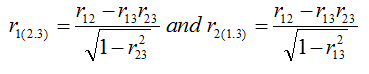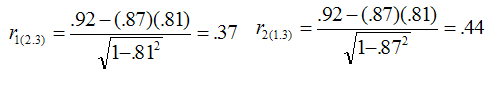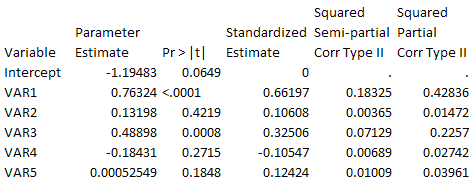# Partial and Semipartial Correlation

Partial correlation measures linear relationship between two variables, while controlling the effect of one or more variable. In this tutorial, we will see the difference between partial and semipartial correlation and how these statistical metrics are calculated mathematically. Also we will cover how it is used in regression analysis.

What is Partial Correlation?

Partial correlation explains the correlation between two continuous variables (let's say X1 and X2) holding X3 constant for both X1 and X2.

Partial Correlation Mathematical Formula

In this case, r12.3 is the correlation between variables x1 and x2 keeping x3 constant. r₁3 is the correlation between variables x1 and x3.Partial Correlation

Let's take an example -

Suppose we want to see the relationship between sales and number of high performing employees keeping promotion budget constant. In this case, sales is the variable1 and high performing employees is the variable 2 and promotion budget the variable3.Formula : Compute Partial Correlation
Examples

1. Relationship between demand of coffee and tea keeping prices of tea controlled.
2. Relationship between GMAT score and number of hours studied keeping SAT score constant.
3. Relationship between weight and number of meals intake while controlling age
4. Relationship between bank deposits and interest rate keeping household rate constant.

What is Semipartial Correlation

Semipartial correlation measures the strength of linear relationship between variables X1 and X2 holding X3 constant for just X1 or just X2. It is also called part correlation.Semipartial Correlation
In the above image,  r1(2.3) means the semipartial correlation between variables X1 and X2 where X3 is constant for X2.Semi Partial Correlation Score

Difference between Partial and Semipartial Correlation
Partial correlation holds variable X3 constant for both the other two variables. Whereas, Semipartial correlation holds variable X3 for only one variable (either X1 or X2). Hence, it is called 'semi'partial.
Assumptions : Partial and Semipartial Correlation

1. Variables should be continuous in nature. For example, weight, GMAT score, sales etc
2. There should be linear relationship between all the three variables. If a variable has non-linear relationship, transform it or ignore the variable.
3. There should be no extreme values (i.e outliers). If outliers are present, we need to treat them either by percentile capping or remove the outlier observations
4. Variables you want to hold constant can be one or more than one

SAS Code : Partial Correlation Coefficient

In this example, we are checking association between height and weight keeping age constant.
PROC CORR data=sashelp.class;
Var Height;
With weight;
Partial age;
Run;Partial Correlation Coefficient

The partial correlation coefficient between weight and height is 0.70467 holding age constant. The p-value for the coefficient is 0.0011. It means we can reject the null hypothesis and concludes that coefficient is significantly different from zero.

R Script : Partial Correlation Coefficient
library(ppcor)
# Partial correlation between "height" and "weight" given "age"
with(mydata, pcor.test(Height,Weight,Age))

R Script : Semipartial Correlation Coefficient

Semi partial correlation - Age constant for Weight only
with(mydata, spcor.test(Height,Weight,Age))
Output
estimate    p.value statistic  n gp  Method
0.4118409 0.08947395  1.807795 19  1 pearson

The estimate value is Pearson Semipartial correlation coefficient.

Semi partial correlation coefficient - Age constant for Height only
with(mydata, spcor.test(Weight,Height,Age))
estimate    p.value statistic  n gp  Method

1 0.4732797 0.04727912  2.149044 19  1 pearson

Squared Partial and Semipartial Correlation

In regression, squared partial and squared semipartial correlation coefficients are used.

Squared partial correlation tells us how much of the variance in dependent variable (Y) that is not explained by variable X2 but explained by X1. In other words, it is the proportion of the variation in dependent variable that was left unexplained by other predictors / independent variables but has been explained by independent variable X1.Squared Partial and Semipartial Correlation

Here, R²y.12 is the r-squared from the regression model in which X1 and X2 are independent variables.

Squared Semi-partial correlation tells us how much of the unique contribution of an independent variable to the total variation in dependent variable. In other words, it explains increment in R-square when an independent variable is added.

Squared Partial correlation will always be greater than or equal to squared semi-partial correlation.
Squared Partial Correlation >= Squared Semi-partial Correlation

SAS Code  : Squared Partial and Semi-Partial Correlation

In PROC REG, the PCORR2 option tells SAS to produce squared-partial correlation and SCORR2 option tells SAS to produce squared semi-partial correlation. The STB option is used to generate standardized estimate and TOL is used to calculate tolerance.
Model Overall = VAR1 - VAR5 / SCORR2 PCORR2 STB TOL ;
run;Regression Output

The squared semi-partial correlation between Overall and VAR1 tells us model R-square is added by 0.18325 if  VAR1 is included in the model.

The squared partial correlation between Overall and VAR1 tells us the proportion of variance in Overall that is not explained by the other independent variables, 43% is explained by VAR1.

Which indicates variable importance?

Squared Semipartial correlation indicates variable importance because it measures incremental value in R-Square. We can rank variables based on high to low values of squared semipartial correlation score.

Relationship between Squared Semipartial correlation and Standardized Estimate
Squared Semipartial Correlation = (Standardized Estimate)² * Tolerance

Can individual squared semi-partial correlation add to R-squared?

Answer is NO. It is because the total variation in dependent variable also constitutes a portion that is due to within correlation between two independent variables.Related PostsDeepanshu founded ListenData with a simple objective - Make analytics easy to understand and follow. He has over 8 years of experience in data science. During his tenure, he has worked with global clients in various domains like Banking, Insurance, Telecom and Human Resource.

12 Responses to "Partial and Semipartial Correlation"
1.what is difference between correlation and partial correlation ?

2.when you are talking about just 2 variables then correlation comes but if you are talking about more than 2 variables then partial correlation comes into picture. So,basically partial correlation is also between 2 variables but controlling the effect of other variables

3.This is very good company for buying Facebook followers, likes. Comments and other social services there service is so good i also buy and happy to deal him.

4.what is difference between correlation and partial correlation ?

5.Amazing article wrote by author. He cleared everything regarding correlation and partial correlation

6.Hey Thanks Author for Sharing Amazing Facts.

7.Keep Sharing Good Content.

8.Awesome Post just land to your site, thanks for sharing.

9.Hey you wrote Wonder full Post, its helps

10.Thanks for sharing such a nice and informative post. I Really appreciate Your work. I am actually enjoy establishing your post.

11.Thanks for sharing such a nice and informative post. I Really appreciate Your work. I am actually enjoy establishing your post.

12.Get social media services at cheap rates buy Instagram followers and likes uk at the lowest price what you need if you will get all things at one place, secure payment via PayPal, money-back guarantee 24/7 customer support. Buy Instagram Followers uk Buy Instagram Likes Uk

Next → ← Prev
Love this Post? Spread the Word!
Share